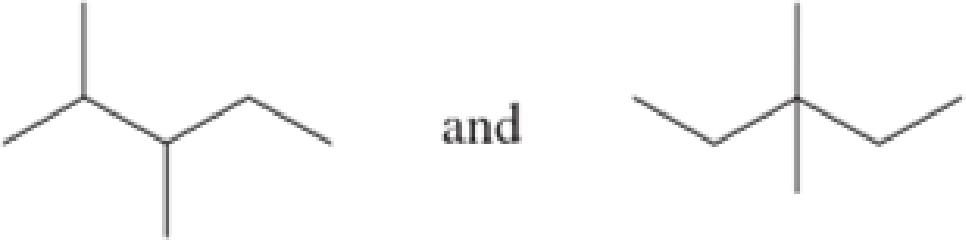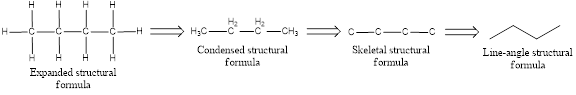Chapter 1, Problem 1.68EP### Organic And Biological Chemistry

7th Edition
STOKER + 1 other
ISBN: 9781305081079

#### Solutions

Chapter
Section### Organic And Biological Chemistry

7th Edition
STOKER + 1 other
ISBN: 9781305081079
Textbook Problem

# Do the line-angle structural formulas in each of the following sets represent (1) the same compound, (2) constitutional isomers, or (3) different compounds that are not constitutional isomers?a.b.(a)

Interpretation Introduction

Interpretation:

Whether the given line-angle structural formulas are same compound, constitutional isomers, or different compound that are not constitutional isomers has to be found.

Concept Introduction:

Organic compounds are represented shortly by the molecular formula and structural formula.  Each and every compound has its own molecular formula.  Compounds can have same molecular formula but not same structural formula.

Isomers are the compounds that have same molecular formula but different structural formula.  The main difference lies in the way the atoms are arranged in the structure.  Isomers have different chemical and physical properties even when they have same molecular formula.  This is known as Isomerism.

If there is difference only in the connectivity of the atoms in the molecule, then it is known as constitutional isomerism.  The isomers are known as constitutional isomers.  They will have same molecular formula and same functional group, but they differ in the connectivity between the atoms in the molecule.

Continuous-chain alkane is the one in which all the carbon atoms are bonded in a linear chain (non-branching chain).

Branched-chain alkane is an alkane which has atleast one branch in its structure that is attached to the continuous chain of carbon atoms.

The structural representation of organic compound can be done in 2D and 3D.  In two-dimensional representation, there are four types of representation in which an organic compound can be drawn.  They are,

• Expanded structural formula
• Condensed structural formula
• Skeletal structural formula
• Line-angle structural formula

Structural formula which shows all the atoms in a molecule along with all the bonds that is connecting the atoms present in the molecule is known as Expanded structural formula.

Structural formula in which grouping of atoms are done and in which the central atoms along with the other atoms are connected to them are treated as group is known as Condensed structural formula.

Structural formula that shows the bonding between carbon atoms alone in the molecule ignoring the hydrogen atoms being shown explicitly is known as Skeletal structural formula.

Structural formula where a line represent carbon‑carbon bond and the carbon atom is considered to be present in each point and the end of lines is known as Line-angle structural formula.Explanation

Given structures are,

The molecular formula for first structure is C7H16

(b)

Interpretation Introduction

Interpretation:

Whether the given line-angle structural formulas are same compound, constitutional isomers, or different compound that are not constitutional isomers has to be found.

Concept Introduction:

Organic compounds are represented shortly by the molecular formula and structural formula.  Each and every compound has its own molecular formula.  Compounds can have same molecular formula but not same structural formula.

Isomers are the compounds that have same molecular formula but different structural formula.  The main difference lies in the way the atoms are arranged in the structure.  Isomers have different chemical and physical properties even when they have same molecular formula.  This is known as Isomerism.

If there is difference only in the connectivity of the atoms in the molecule, then it is known as constitutional isomerism.  The isomers are known as constitutional isomers.  They will have same molecular formula and same functional group, but they differ in the connectivity between the atoms in the molecule.

Continuous-chain alkane is the one in which all the carbon atoms are bonded in a linear chain (non-branching chain).

Branched-chain alkane is an alkane which has atleast one branch in its structure that is attached to the continuous chain of carbon atoms.

The structural representation of organic compound can be done in 2D and 3D.  In two-dimensional representation, there are four types of representation in which an organic compound can be drawn.  They are,

• Expanded structural formula
• Condensed structural formula
• Skeletal structural formula
• Line-angle structural formula

Structural formula which shows all the atoms in a molecule along with all the bonds that is connecting the atoms present in the molecule is known as Expanded structural formula.

Structural formula in which grouping of atoms are done and in which the central atoms along with the other atoms are connected to them are treated as group is known as Condensed structural formula.

Structural formula that shows the bonding between carbon atoms alone in the molecule ignoring the hydrogen atoms being shown explicitly is known as Skeletal structural formula.

Structural formula where a line represent carbon‑carbon bond and the carbon atom is considered to be present in each point and the end of lines is known as Line-angle structural formula.### Still sussing out bartleby?

Check out a sample textbook solution.

See a sample solution

#### The Solution to Your Study Problems

Bartleby provides explanations to thousands of textbook problems written by our experts, many with advanced degrees!

Get Started